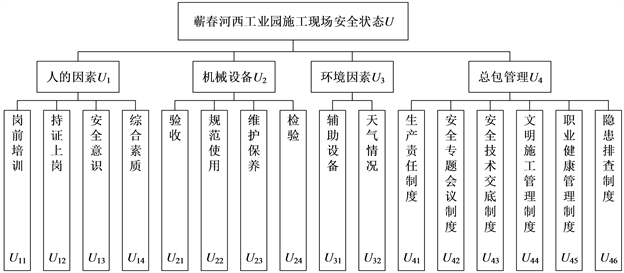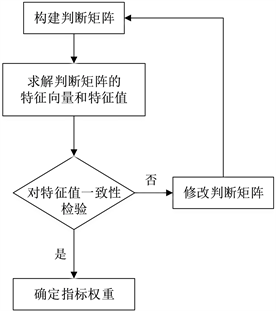﻿ 基于AHP-FUZZY施工现场安全评价研究

# 基于AHP-FUZZY施工现场安全评价研究Research on Safety Evaluation of Construction Site Based on AHP-FUZZY

Abstract: Aiming at the problems of safety accidents and the seriousness of losses in the construction industry, this paper introduces the fuzzy analytic hierarchy process (AHP-FUZZY) and establishes a safety evaluation model based on AHP-FUZZY. The weight of each evaluation index of the safety evaluation model is determined by AHP method, and the comprehensive safety evaluation of the construction site of Qichun Hexi Industrial Park is carried out in combination with the fuzzy mathematics theory. The construction site is in a basically normal safety state, which is consistent with the actual situation. This method is of great significance for improving the level of enterprise safety management.

1. 引言

2. AHP-FUZZY安全评价模型

2.1. 建立安全评价指标体系

2.2. 确定评语集Figure 1. Qichun Hexi Industrial Park safety evaluation index systemTable 1. Construction site safety and safety evaluation index classification

2.3. 确定评价指标模糊隶属度

$R=\left(\begin{array}{cccc}{r}_{11}& {r}_{12}& \cdots & {r}_{1n}\\ {r}_{21}& {r}_{22}& \cdots & {r}_{2n}\\ ⋮& ⋮& \ddots & ⋮\\ {r}_{m1}& {r}_{m2}& \cdots & {r}_{mn}\end{array}\right)$

2.4. 构造判断矩阵

2.5. 确定指标权重Table 2. Judgment matrix scale meaningFigure 2. Indicator weight determination process

3. 实例分析

3.1. 工程概况

3.2. 构建判断矩阵Table 4. U-Ui, Ui-Uij judgment matrix

3.3. 确定评价指标权重

3.4. 确定评价指标模糊隶属度

3.5. 模糊综合评价

${B}_{1}=\left(0.101,0.267,0.119,0.513\right)×{R}_{1}=\left(0.266,0.442,0.241,0.051,0\right)$

${B}_{2}=\left(0.059,0.431,0.399,0.111\right)×{R}_{2}=\left(0.336,0.463,0.145,0.056,0\right)$

${B}_{3}=\left(0.833,0.167\right)×{R}_{3}=\left(0.517,0.350,0.108,0.025,0\right)$

${B}_{4}=\left(0.144,0.053,0.157,0.297,0.311,0.038\right)×{R}_{4}=\left(0.484,0.453,0.059,0.004,0\right)$

$B=\left({B}_{1},{B}_{2},{B}_{3},{B}_{4}\right)=\left(\begin{array}{ccccc}0.266& 0.442& 0.241& 0.051& 0.000\\ 0.336& 0.463& 0.145& 0.056& 0.000\\ 0.517& 0.350& 0.108& 0.025& 0.000\\ 0.484& 0.453& 0.059& 0.004& 0.000\end{array}\right)$

$S=\left(0.293,0.137,0.048,0.523\right)×B=\left(0.401,0.447,0.127,0.025,0\right)$

4. 结论

NOTES

*通讯作者。

 袁春燕, 杨剑耕, 卢俊龙. 模糊测度理论在施工现场安全目标决策中的应用[J]. 中国安全生产科学技术, 2014, 10(3): 101-105.

 向鹏成, 陈远方. 建筑工程施工现场安全性评价及预警研究[J]. 项目管理技术, 2016, 14(9) :50-55.

 陈娟娟. 基于可拓云理论的施工安全综合评价及应用研究[D]: [硕士学位论文]. 武汉: 武汉科技大学, 2017.

 单仁亮, 宁海龙, 李健芳. 基于组合赋权法的建筑施工现场安全综合评价[J]. 沈阳建筑大学学报(社会科学版), 2018, 20(2): 168-175.

 尹传根, 侯学良. 基于组合赋权法的特高压输变电工程施工质量灰色评价模型[J]. 河南工程学院学报(自然科学版), 2017, 29(3): 53-59.

 张仕廉, 许海鸿. 建筑施工现场组织及员工安全行为评价[J]. 建筑经济, 2017, 38(1): 53-57.

 黄世国. 建筑施工现场安全综合评价体系研究[D]: [硕士学位论文]. 重庆: 重庆大学, 2007.

 董秋实. 建筑施工现场安全评价体系的研究[D]: [硕士学位论文]. 哈尔滨: 哈尔滨工业大学, 2009.

 曹红峰. 建筑施工现场安全评价体系相关问题探讨[J]. 黑龙江科学, 2015, 6(6): 107.

 中华人民共和国建设部. 施工企业安全生产评价标准(JGJ/T77-2010) [S]. 北京: 中国建筑工业出版社, 2003.

Top